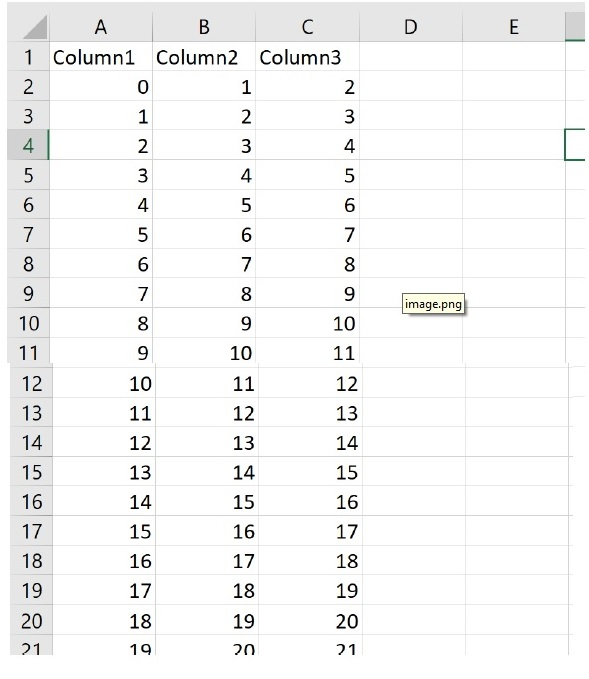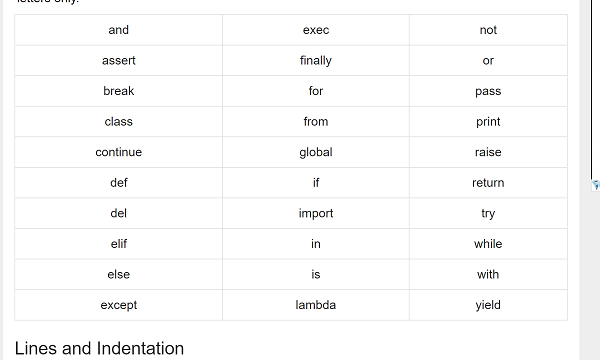How to save HTML Tables data to CSV in Python

PythonServer Side ProgrammingProgrammingHTML

Problem:

One of the most challenging taks for a data sceintist is to collect the data. While the fact is, there is plenty of data available in the web it is just extracting the data through automation.

Introduction..

I wanted to extract the basic operations data which is embedded in HTML tables from https://www.tutorialspoint.com/python/python_basic_operators.htm.

Hmmm, The data is scattered in many HTML tables, if there is only one HTML table obviously I can use Copy & Paste to .csv file.

However, if there are more than 5 tables in a single page then obviously it is pain. Isn't it ?

How to do it..

1. I will quickly show you how to create an csv file easily if you want to create a csv file.

import csv
# Open File in Write mode , if not found it will create one
File = open('test.csv', 'w+')
Data = csv.writer(File)

Data.writerow(('Column1', 'Column2', 'Column3'))

# Write data
for i in range(20):
Data.writerow((i, i+1, i+2))

# close my file
File.close()

Output

The above code when executed produces a test.csv file with in the same directory as this code.2. Let us now retrieve an HTML table from https://www.tutorialspoint.com/python/python_dictionary.htm and write it as a CSV file.

First step is to do imports.

import csv
from urllib.request import urlopen
from bs4 import BeautifulSoup
url = 'https://www.tutorialspoint.com/python/python_dictionary.htm'
• Open the HTML file and store it in html object using urlopen.

Output

html = urlopen(url)
soup = BeautifulSoup(html, 'html.parser')
• Find the tables inside the html table and Let us bring the tables data. For demonstration purpose I will be extracting only the first table 

Output

table = soup.find_all('table')
rows = table.find_all('tr')

print(rows)

Output

[<tr>
<th style='text-align:center;width:5%'>Sr.No.</th>
<th style='text-align:center;width:95%'>Function with Description</th>
</tr>,
<tr>
<td class='ts'>1</td>
<td><a href='/python/dictionary_cmp.htm'>cmp(dict1, dict2)</a>
<p>Compares elements of both dict.</p></td>
</tr>, <tr>
<td class='ts'>2</td>
<td><a href='/python/dictionary_len.htm'>len(dict)</a>
<p>Gives the total length of the dictionary. This would be equal to the number of items in the dictionary.</p></td>
</tr>,
<tr>
<td class='ts'>3</td>
<td><a href='/python/dictionary_str.htm'>str(dict)</a>
<p>Produces a printable string representation of a dictionary</p></td>
</tr>,
<tr>
<td class='ts'>4</td>
<td><a href='/python/dictionary_type.htm'>type(variable)</a>
<p>Returns the type of the passed variable. If passed variable is dictionary, then it would return a dictionary type.</p></td>
</tr>]

5. Now we will write the data to csv file.

Example

File = open('my_html_data_to_csv.csv', 'wt+')
Data = csv.writer(File)
try:
for row in rows:
FilteredRow = []
for cell in row.find_all(['td', 'th']):
FilteredRow.append(cell.get_text())
Data.writerow(FilteredRow)
finally:
File.close()

6. The results are now saved into my_html_data_to_csv.csv file.

Example

We will put everything explained above together.

Example

import csv
from urllib.request import urlopen
from bs4 import BeautifulSoup

# set the url..
url = 'https://www.tutorialspoint.com/python/python_basic_syntax.htm'

# Open the url and parse the html
html = urlopen(url)
soup = BeautifulSoup(html, 'html.parser')

# extract the first table
table = soup.find_all('table')
rows = table.find_all('tr')

# write the content to the file
File = open('my_html_data_to_csv.csv', 'wt+')
Data = csv.writer(File)
try:
for row in rows:
FilteredRow = []
for cell in row.find_all(['td', 'th']):
FilteredRow.append(cell.get_text())
Data.writerow(FilteredRow)
finally:
File.close()

Table in the html page.